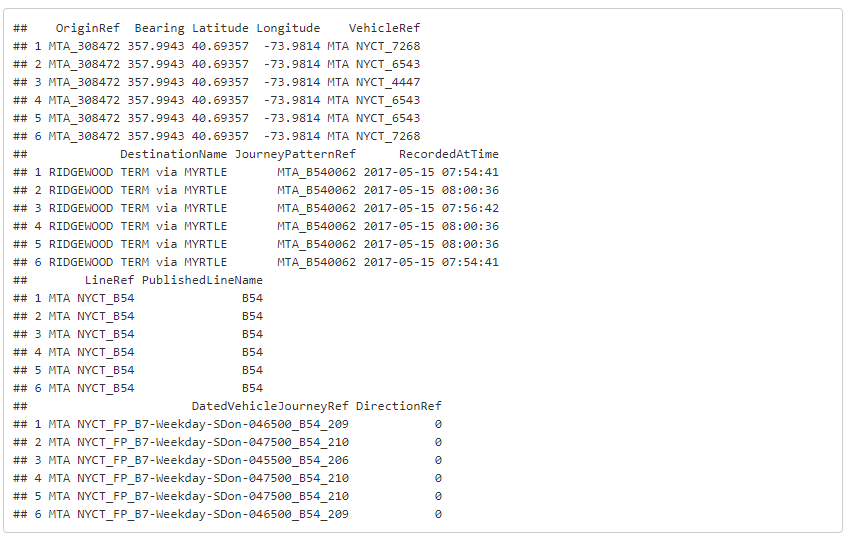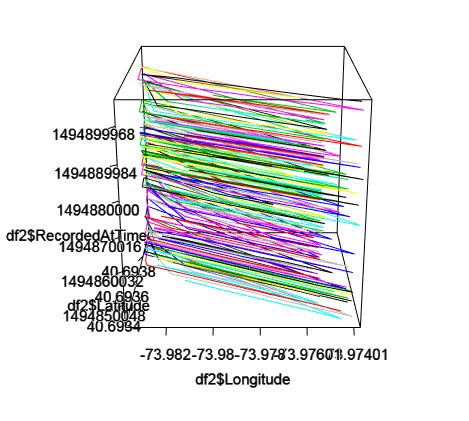# Creating a 3D Time-Space Diagram using R— MTA BusTime Data

`library("rgl")`
`# Read the input into a dataframe df <- read.csv("../Data/pings.csv", head=TRUE, skip=1) # Check the class of the RecordedAtTime column class(df\$RecordedAtTime)##  "factor"# convert the class of the RecordedAtTime column df\$RecordedAtTime <- as.POSIXlt(df\$RecordedAtTime) # Extract hour from the column head(df\$RecordedAtTime\$hour)##  7 8 7 8 8 7`
`# Create another dataframe which will contain one bus line and one direction # Bus line = B54 df2 <- subset(df, df\$PublishedLineName == "B54") # Direction = 0 df2 <- subset(df2, df2\$DirectionRef == 0) # Let's take a look at the data head(df2)`First 6 rows of the dataframe
`# Before creating a 3D Plot, we need to order the dataframe by RecordedAtTime df2 <- df2[with(df2, order(df2\$RecordedAtTime)),] # This function will create an interactive 3D plot plot3d(df2\$Longitude,df2\$Latitude, df2\$RecordedAtTime, type="l", col=as.numeric(df2\$DatedVehicleJourneyRef)) rglwidget()`The interactive plot can be seen at http://www.tandoncsmart.com/week3.php

--

--

## More from Abdullah Kurkcu

Lead Traffic Modeler @Ulteig

Love podcasts or audiobooks? Learn on the go with our new app.